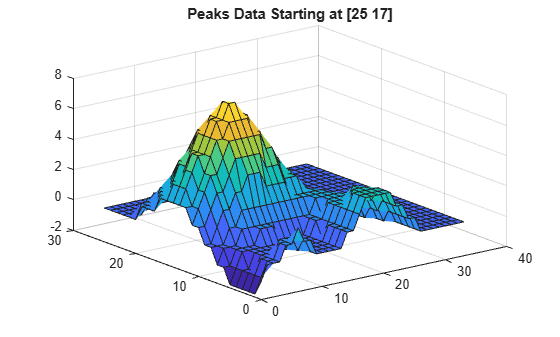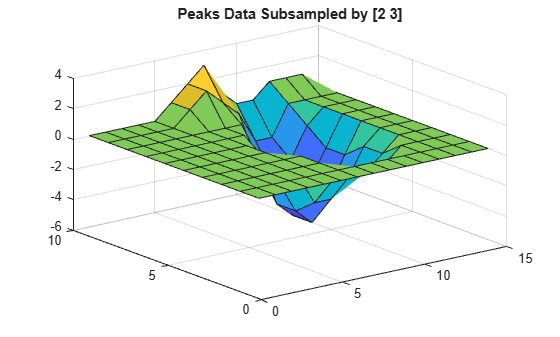Read data from variable in netCDF data source

## Syntax

``vardata = ncread(source,varname)``
``vardata = ncread(source,varname,start,count)``
``vardata = ncread(source,varname,start,count,stride)``

## Description

example

````vardata = ncread(source,varname)` reads all the data from the netCDF variable `varname` contained in `source`. The returned variable value `vardata` is of the MATLAB® data type that best matches the netCDF data type of `varname`.```

example

````vardata = ncread(source,varname,start,count)` reads data beginning at the location specified by `start`. The `count` argument specifies the number of elements to read along each dimension.```

example

````vardata = ncread(source,varname,start,count,stride)` returns data with the interval specified by `stride` between the indices of each dimension of the variable.```

## Examples

collapse all

Read and plot a variable named `peaks` from the netCDF file `example.nc`.

```peaksData = ncread("example.nc","peaks"); whos peaksData```
``` Name Size Bytes Class Attributes peaksData 50x50 5000 int16 ```

Plot `peaksData` and add a title.

```surf(peaksData) title("Peaks Data")```Read and plot only a subset of the `peaks` variable data starting from the location `[25 17]` until the end of each dimension.

```start = [25 17]; % Start location along each coordinate count = [Inf Inf]; % Read until the end of each dimension peaksData = ncread("example.nc","peaks",start,count); whos peaksData```
``` Name Size Bytes Class Attributes peaksData 26x34 1768 int16 ```

Plot the data.

```surf(peaksData) title("Peaks Data Starting at [25 17]")```Read and plot data, where the data is sampled at a specified spacing between variable indices along each dimension. Read variable data at intervals specified in `stride`, starting from the location in `start`. A value of `1` in `stride` accesses adjacent values in the corresponding dimension, a value of `2` accesses every other value in the corresponding dimension, and so on.

```start = [1 1]; count = [10 15]; stride = [2 3]; sampledPeaksData = ncread("example.nc","peaks",start,count,stride); whos sampledPeaksData```
``` Name Size Bytes Class Attributes sampledPeaksData 10x15 300 int16 ```

Plot the data.

```surf(sampledPeaksData) title("Peaks Data Subsampled by [2 3]")```## Input Arguments

collapse all

Name of the netCDF data source, specified as a string scalar or character vector. The `source` argument can be one of these values:

• The path of a local netCDF source

• The OPeNDAP URL of a remote OPeNDAP netCDF data source

• The HTTP URL of a remote netCDF source, with `#mode=bytes` appended to the end of the URL to enable byte-range reading

Note

Example: `"myNetCDFfile.nc"`

Example: `"http://host_name/netcdf_filename#mode=bytes"`

Variable name, specified as a string scalar or character vector containing the name of a variable in the netCDF data source.

If `source` specifies a file with format `netcdf4`, you can specify the location of the variable within the group hierarchy by specifying `varname` as a fully qualified name.

Example: `"myVar"`

Example: `"/myGrp/mySubGrp/myNestedVar"`

Starting location of the data in the variable, specified as a numeric vector of positive integers. For an `N`-dimensional variable, specify `start` as a vector of length `N` containing 1-based indices.

If you do not specify `start`, then the `ncread` function starts reading the variable from the first index along each dimension.

Example: `[2 1 3]`

Data Types: `double`

Number of elements to read, specified as a numeric vector of positive integers or `Inf` values. For an `N`-dimensional variable, specify `count` as a vector of length `N`, containing the number of elements to read along each dimension. If any element of `count` is `Inf`, then `ncread` reads until the end of the corresponding dimension.

If you do not specify `count`, then the `ncread` function reads the variable data until the end of each dimension.

Example: `[Inf 10 50]`

Data Types: `double`

Space between the variable indices along each dimension, specified as a numeric vector of integers. For an `N`-dimensional variable, specify `stride` as a vector of length `N`. The elements of the `stride` vector correspond, in order, to the dimensions of the variable. A value of `1` accesses adjacent values of the netCDF variable in the corresponding dimension, a value of `2` accesses every other value in the corresponding dimension, and so on.

If you do not specify `stride`, then the `ncread` function reads the data with a default spacing of `1` along each dimension.

Example: `[2 10 1]`

Data Types: `double`

## Output Arguments

collapse all

Variable data, returned as a numeric array, text, or cell array of the MATLAB data type that best matches the netCDF data type of `varname`. For more information about how MATLAB determines the best match, see NetCDF to MATLAB Data Type Conversion.

For numeric variables, when at least one of the attributes `_FillValue`, `scale_factor`, or `add_offset` is present, `vardata` is of type `double`. Additionally, `ncread` applies these attribute conventions in a sequence before returning `vardata`:

1. If the `_FillValue` attribute exists:

• If `vardata` is of type `double` or `single`, then `ncread` replaces `vardata` values equal to that of the `_FillValue` attribute with `NaN` values.

• If `vardata` is of any other numeric type, then `ncread` replaces `NaN` `vardata` values as well as `vardata` values equal to that of the `_FillValue` attribute with `0` values.

2. If the `scale_factor` attribute exists, then `ncread` multiplies the values in `vardata` by the value of the `scale_factor` attribute.

3. If the `add_offset` attribute exists, then `ncread` adds the value of the `add_offset` attribute to the values in `vardata`.

Note

If `varname` is of type `NC_STRING`, then it can contain UTF-8-encoded characters; if `varname` is of type `NC_CHAR`, then it must contain only ASCII-encoded characters.

Data Types: `single` | `double` | `int8` | `int16` | `int32` | `int64` | `uint8` | `uint16` | `uint32` | `uint64` | `cell` | `char` | `string`

collapse all

### NetCDF to MATLAB Data Type Conversion

The netCDF-related MATLAB functions automatically choose the MATLAB data type that best matches the netCDF data type according to this table.

NetCDF Data TypeMATLAB Data Type
`NC_DOUBLE``double`
`NC_FLOAT``single`
`NC_INT``int32`
`NC_SHORT``int16`
`NC_BYTE``int8`
`NC_CHAR``char`
`NC_STRING` (*)`string`
`NC_INT64` (*)`int64`
`NC_UINT64` (*)`uint64`
`NC_UINT` (*)`uint32`
`NC_USHORT` (*)`uint16`
`NC_UBYTE` (*)`uint8`
User-defined `NC_VLEN` types (*)`cell`

(*) These netCDF data types are available only for files with format `netcdf4`.

## Tips

• MATLAB interprets multidimensional data as column-major, but the netCDF C API interprets multidimensional data as row-major. Multidimensional data in the netCDF C API shows dimensions in the reverse of the order shown by MATLAB and consequently appears transposed.

## Version History

Introduced in R2011a

expand all